# Maths - frame-of-reference for combining rotations.

When we are modelling rotation it is important to consider the frame of reference. In the following we will use the example of an aeroplane, to a person on the ground the aeroplane might be turning right, but to a person in the aeroplane the ground will appear to be turning left. So the orientation of the plane relative to the ground is minus the orientation of the ground relative to the plane.

But the situation gets more complicated when we are trying to work out the result of a sequence of rotations, I can think of two possible ways to combine two rotations. In the first case we used the fixed global co-ordinates for everything, in the second case we specify the second rotation in the local co-ordinates of the aeroplane, which have been rotated by the first rotation.

Note: the following assumes:

The following examples show two possible ways to combine rotations:

## Global frame-of-reference

always defines rotations in global coordinates, in this case x=right, y=up, z=toward viewer. In this case the matrix or quaternion representations of the individual rotations can be combined by multipling in the following order:

[resulting transform] = [second transform] * [first rotation]

To see why this is we could multiply vector Vin by matrix [A] to produce an intermediate vector Vmid, we then multipy this by matrix [B]. If we sustitute [A]Vin for Vmid we can see that the overall matrix = [B][A].## Local frame-of-reference

Defines rotations relative to local coordinates, in other words the coordinate system rotates with the object. In this case the matrix or quaternion representations of the individual rotations can be combined by multipling in the following order:

[resulting transform] = [first rotation] * [second transform]

The easiest way to work in this situation is, instead of imagining ourselves looking at the object from outside and calculating its coodinates, instead we imagine ourselves sitting on the object and measure the coordinates of external points. This means that instead of working in terms of the objects position transform, we are working in terms of its inverse (which is the transpose for rotations as explained here). We can now calculate the result of consecutive transforms by multipling the individual transforms as we did before. Normally when we multiply two matricies, we calculate an element by combinig the corresponding row in the first matrix by the corresponding column in the second matrix. However since the matricies are transposed this is equivilant to reversing the order of matricies from the global case. This forms an isomorphism as shown here:## Which to use

The Global frame of reference is easiest for us to understand and it makes sense when we are moving objects by hand one step at a time. However, for real world objects, such as aeroplane or a robot arm the first rotation tends to alter the coordinate reference for susequent rotations. Therefore the local frame of reference might be used more often.

## Example

Here are a set of rotations in global and local for comparison:
Global Frame-of-Reference Local Frame-of-Reference

Initial Orientation:Initial Orientation:First rotate about y (in global frame) through +90 degrees to give:0 0 1 0 1 0 -1 0 0

First rotate about y (in local frame which is the same as global frame at this stage) through +90 degrees to give:0 0 1 0 1 0 -1 0 0

Note that the local co-ordinates are rotated with the aeroplane

Now rotate about z through +90 degrees.resulting matrix
 0 -1 0 0 0 1 -1 0 0
= rotate +90 about absolute z
(this rotation)
 0 -1 0 1 0 0 0 0 1
(first rotation)
 0 0 1 0 1 0 -1 0 0

Now rotate about z in the updated local co-ordinates through +90 degrees.resulting matrix
 0 0 1 1 0 0 0 1 0
(first rotation)
 0 0 1 0 1 0 -1 0 0
* rotate +90 about local z
(this rotation)
 0 -1 0 1 0 0 0 0 1

Now rotate about y through -90 degrees.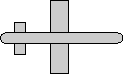resulting matrix
 1 0 0 0 0 1 0 -1 0
= rotate -90 about absolute y
(this rotation)
 0 0 -1 0 1 0 1 0 0
* result from above
 0 -1 0 0 0 1 -1 0 0

So the final result is equivalent to rotating about the x axis (even though none of the individual rotations were about the x axis)

Now rotate about y in the updated local co-ordinates through -90 degrees.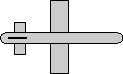resulting matrix
 1 0 0 0 0 -1 0 1 0
=

result from above

 0 0 1 1 0 0 0 1 0
* rotate -90 about absolute y
(this rotation)
 0 0 -1 0 1 0 1 0 0

I have tried to think of a mechanism where we would use each of these two possible schemes, and the following is the best that I can come up with: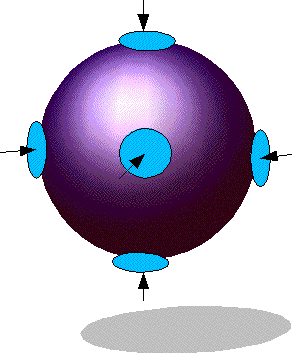The best model I can think of here is a system which rotates a ball with a pair of suckers on each axis, each pair of suckers can turn the ball but otherwise will slide over it (it has rotational but not linear friction).. This does not seem like a very practical situation and therefore it is less important to model it, but I think this is important when we start to think about the physics because we often want to calculate the rotational quantities relative to the inertial frame which is global. I would be interested to hear if anyone could work out the mathematics that would allow use to combine rotations in this situation.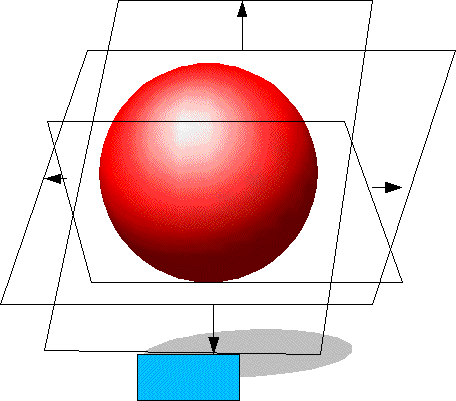This model is like a gimbol that might hold a gyroscope. Here the ball is allowed to rotate in one plane relative to a frame, the frame itself is allowed to rotate in another plane relative to an outer frame, and so on. This situation can easily be modelled using a sequence of matrix or quaternion multiplications. Each multiplication takes us to the next frame inside the last one. This is the same as a jointed structure such as a robot arm, except that most jointed structures will have linear as well as rotational movement, and so we must use affine instead of orthogonal transforms, however the principles are the same, so this is an important situation to model in practice.

 metadata block see also: Correspondence about this page Book Shop - Further reading. Where I can, I have put links to Amazon for books that are relevant to the subject, click on the appropriate country flag to get more details of the book or to buy it from them.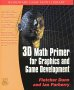3D Math Primer - Aimed at complete beginners to vector and matrix algebra. Other Math Books

This site may have errors. Don't use for critical systems.